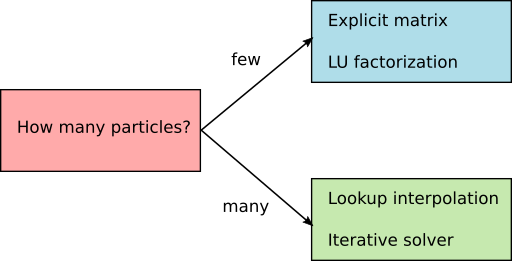# Solver settings¶

Note

This section is relevant if you want to simulate systems with many particles

In order to limit the runtime, Smuthi currently offers two numerical strategies for the solution of the scattering problem:

1. LU factorization, that is basically a variant of Gaussian elimination. To this end, the interaction matrix is fully stored in memory.
2. Iterative solution with the GMRES method. In this case, you can either store the full interaction matrix in memory, or use a lookup from which the matrix entries are approximated by interpolation, see Amos Egel’s PhD thesis (section 3.10.1) or [Egel, Kettlitz, Lemmer, 2016]

With growing particle number, all involved operations get more expensive, but the costs of LU factorization grow faster than the cost of iterative solution. Similarly, costs of calculating of the full interaction matrix grows faster than the cost of computing a lookup table. For this reason, we recommend the following decision scheme:The numerical strategy for solving the linear system is defined through the input parameters of the simulation constructor. The relevant parameters are:

• solver_type: Either “LU” or “gmres”
• solver_tolerance: This parameter defines the abort criterion. If the residual is smaller than the tolerance, the solver halts. The parameter is ignored in case of “LU” solver type.
• store_coupling_matrix: If true, the coupling matrix is explicitly calculated and stored in memory. Otherwise, a lookup table is prepared and the matrix-vector multiplications are run on the fly, where the matrix entries are computed using the lookup table. The parameter is ignored in case of “LU” solver type.
• coupling_matrix_lookup_resolution: If lookup tables should be used, this needs to be set to a distance value that defines the spatial resolution of the lookup table. The parameter is ignored when the coupling matrix is explicitly calculated.
• coupling_matrix_interpolator_kind: If lookup tables should be used, define here either “linear” or “cubic” interpolation. “linear” is faster and “cubic” is more precise for the same resolution. The parameter is ignored when the coupling matrix is explicitly calculated.

This would be a typical setting for a small number of particles:

simulation = smuthi.simulation.Simulation( ...
solver_type='LU',
store_coupling_matrix=True,
... )


This would be a typical setting for a large number of particles:

simulation = smuthi.simulation.Simulation( ...
solver_type='gmres',
solver_tolerance=1e-4,
store_coupling_matrix=False,
coupling_matrix_lookup_resolution=5,
coupling_matrix_interpolator_kind='linear',
... )


Note that GPU acceleration is currently only available for particle coupling through lookup interpolation.